Browse by Topic
Related Topics

# Self-Description

I am a big fan of Randall Munroe’s web comic strip xkcd. (Apparently I am not alone.)

A while ago, Randall posted a strip with a self-referential chart of the amount of black ink in the image.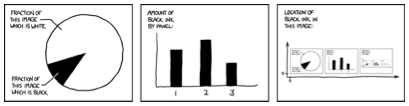If you have read my past blog items, you know I like recursive pictures. So I thought I would create a Mathematica version of this strip.

First, I generated each of the charts, which are all pretty straightforward chart types in Mathematica. The work was in making them roughly follow Randall’s style choices.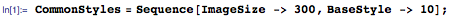The pie chart: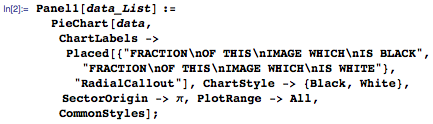The bar chart: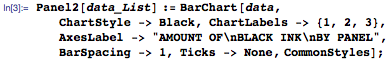The ink location chart:Next, I solved the equation f[x]=x, where f is the function that generates an analysis report on the ink in an image and x is the image of the report.

If the equation is substituted into itself, it also solves f[f[x]]=x, and for that matter f[f[f[f[f[f[f[x]]]]]]]=x.

If f meets certain convergence criteria, then this problem can be solved iteratively just by working out f[f[f[…f[x]…]]] for some random initial guess at x.

This is exactly the numerical analysis task that every bored schoolchild has done at some point by pressing the Cos button on a calculator repeatedly. It eventually converges on 0.739085, which is the solution to the equation Cos[x]=x. In Mathematica this is easily done with: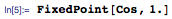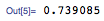So all I needed was a function that generates a cartoon strip based on the analysis of a cartoon strip. I started by creating a measure of black ink in an image. Since Mathematica mostly prefers vector graphics, I first needed to convert to a raster image before I could count pixels.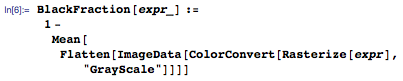And then I fed the results of that into the panel generating functions.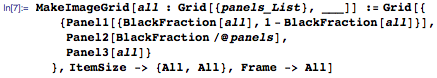I then used it on a completely blank strip as the initial guess.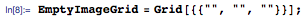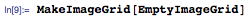Since I started with a blank strip, it registered no black ink. But the second iteration picked up the black from the edge of the pie chart, the frame, the axes, and the text in the first iteration.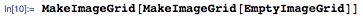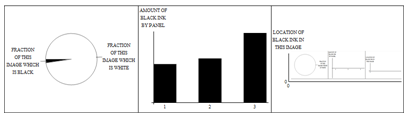Then I did the same as we all did with Cos and found the fixed point, the point at which applying the function again makes no difference.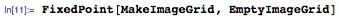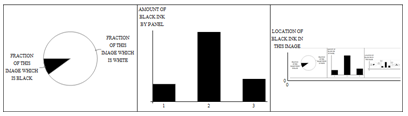I didn’t deal with convergence criteria here, and for some design choices, that is a problem. But in practice, for this task, convergence problems are cycles of dithering of individual anti-aliased pixels, rather than a fundamental divergence. The differences become imperceptible to the human eye after 10 or 20 iterations. So the following is more practical than FixedPoint: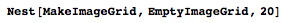One can quickly extend the idea to a color version. Here is one in CMYK (cyan, magenta, yellow, black—the standard ink colors), but one could equally do RGB, HSB, or CMYK+White.

First, I had to update the styles to make the strip colorful.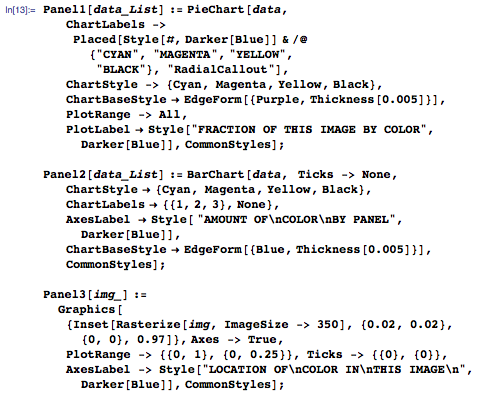Then I needed a new color analysis function and MakeImageGrid function.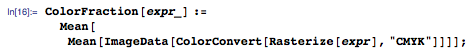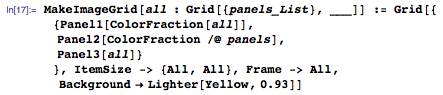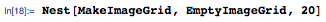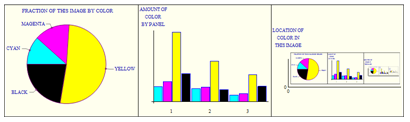If I could just get Mathematica to automate the humor of xkcd, then I would make a fortune!